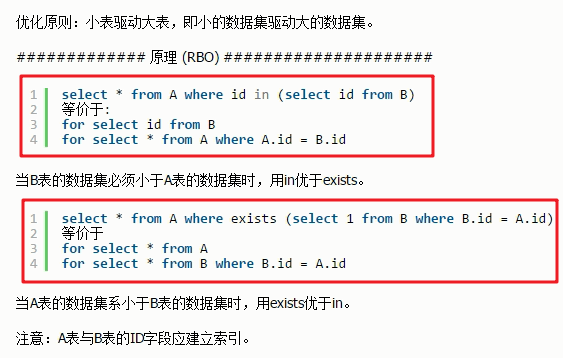### 1.为什么要小表驱动大表呢

for(int i=5;.......)
{
for(int j=1000;......)
{}
}

### 2.数据准备### 3.案例演示

①当B表的数据集小于A表数据集时，用in优于exists。

select *from tb_emp_bigdata A
where A.deptno in (select B.deptno from tb_dept_bigdata B)

B表为tb_dept_bigdata：100条数据，A表tb_emp_bigdata：5000条数据。select *from tb_emp_bigdata A
where exists(select 1 from tb_dept_bigdata B where B.deptno=A.deptno);②当A表的数据集小于B表的数据集时，用exists优于in。

select *from tb_dept_bigdata A
where A.deptno in(select B.deptno from tb_emp_bigdata B);select *from tb_dept_bigdata A
where exists(select 1 from tb_emp_bigdata B where B.deptno=A.deptno);### 4.总结

in后面跟的是小表，exists后面跟的是大表。

select …..from table where exists(subquery);